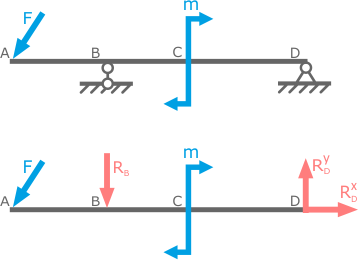Reaction of supports

Supporting reactions arise under the influence of external loads in the supports and keep the element in question or the construction in equilibrium.Replacement of supports by their reactions

When calculating the elements of the reaction, the supports also act as external forces applied to the body under consideration.

The problem of determining the support reactions is considered in detail in the course of theoretical mechanics, but in practice it is often used in solving problems of material resistance.

In this case, some problems in the co-sustainability can be solved without their definition. This is possible in cases where a bar is adopted for the design scheme, fixed in a rigid support (seal) without additional supports, for example, statically determinate cantilever beams, rods or rod systems.

Video by subject (contains English subtitles)

Determination of reactions

The number and direction of reactions depends both on the type of supports and on the method of loading the beam and for statically determinate systems are determined from the equations of equilibrium of the structure or its elements.

Video by subject (contains English subtitles)

For the general case of loading (spatial systems), in which up to 6 support reactions can occur, an appropriate number of equations are required.

For example, from the condition that the given system does not move relative to the supports in space (right-to-left, up-down, and back-to-back), we can equate to zero the sum of the projections of all forces on the x, y and z axes.

∑F(x)=0;
∑F(y)=0;
∑F(z)=0.

From the condition that the system does not rotate, we equate to zero the sums of the moments of all loads with respect to the corresponding axes.

∑m(x)=0;
∑m(y)=0;
∑m(z)=0.

A joint solution of the system of equations obtained makes it possible to determine the magnitude and direction of the reactions in the supports.

For a planar loading system, a maximum of three static equations can be made to determine up to three desired forces in the supports.

Linearly loaded elements allow us to write down only one equation of equilibrium.

To calculate the reactions of supports of statically indeterminate systems, in addition to the equations of statics, additional dependences are required that relate the forces to the deformations corresponding to them.

In some cases, the support reactions can be equal to zero. This only indicates that the external loads and other reactions are mutually balanced in such a way that the system can remain static and without the corresponding force at a given point.